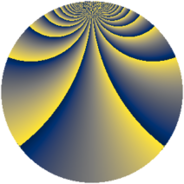# Properties

 Label 1520.2.ckLevel $1520$ Weight $2$ Character orbit 1520.ck Rep. character $\chi_{1520}(501,\cdot)$ Character field $\Q(\zeta_{12})$ Dimension $640$ Sturm bound $480$

# Related objects

## Defining parameters

 Level: $$N$$ $$=$$ $$1520 = 2^{4} \cdot 5 \cdot 19$$ Weight: $$k$$ $$=$$ $$2$$ Character orbit: $$[\chi]$$ $$=$$ 1520.ck (of order $$12$$ and degree $$4$$) Character conductor: $$\operatorname{cond}(\chi)$$ $$=$$ $$304$$ Character field: $$\Q(\zeta_{12})$$ Sturm bound: $$480$$

## Dimensions

The following table gives the dimensions of various subspaces of $$M_{2}(1520, [\chi])$$.

Total New Old
Modular forms 976 640 336
Cusp forms 944 640 304
Eisenstein series 32 0 32

## Trace form

 $$640q + O(q^{10})$$ $$640q + 4q^{10} + 16q^{15} - 4q^{16} + 8q^{19} + 8q^{24} - 32q^{26} - 40q^{28} - 40q^{32} + 28q^{34} - 16q^{36} + 40q^{38} + 40q^{42} + 44q^{44} - 112q^{46} + 80q^{48} - 640q^{49} + 8q^{51} + 32q^{52} + 60q^{54} - 24q^{56} + 56q^{58} - 32q^{61} + 64q^{62} - 48q^{64} - 104q^{66} + 88q^{68} + 64q^{69} - 56q^{72} - 16q^{74} - 96q^{76} - 32q^{79} - 16q^{80} + 320q^{81} + 80q^{82} + 88q^{84} - 16q^{85} - 32q^{86} - 40q^{88} + 36q^{90} + 24q^{92} + 24q^{94} + 456q^{96} - 108q^{98} - 32q^{99} + O(q^{100})$$

## Decomposition of $$S_{2}^{\mathrm{new}}(1520, [\chi])$$ into newform subspaces

The newforms in this space have not yet been added to the LMFDB.

## Decomposition of $$S_{2}^{\mathrm{old}}(1520, [\chi])$$ into lower level spaces

$$S_{2}^{\mathrm{old}}(1520, [\chi]) \cong$$ $$S_{2}^{\mathrm{new}}(304, [\chi])$$$$^{\oplus 2}$$